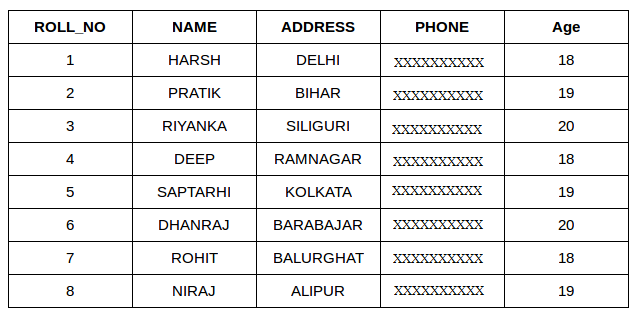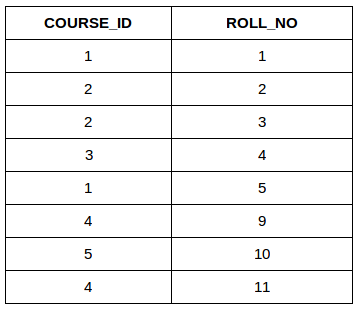# SQL | Join (Inner, Left, Right and Full Joins)

• Difficulty Level : Easy
• Last Updated : 09 Nov, 2020

A SQL Join statement is used to combine data or rows from two or more tables based on a common field between them. Different types of Joins are:

• INNER JOIN
• LEFT JOIN
• RIGHT JOIN
• FULL JOIN

Consider the two tables below:

Attention reader! Don’t stop learning now. Learn SQL for interviews using SQL Course  by GeeksforGeeks.

StudentStudentCourseThe simplest Join is INNER JOIN.

1. INNER JOIN: The INNER JOIN keyword selects all rows from both the tables as long as the condition satisfies. This keyword will create the result-set by combining all rows from both the tables where the condition satisfies i.e value of the common field will be same.
Syntax:
```SELECT table1.column1,table1.column2,table2.column1,....
FROM table1
INNER JOIN table2
ON table1.matching_column = table2.matching_column;

table1: First table.
table2: Second table
matching_column: Column common to both the tables.
```

Note: We can also write JOIN instead of INNER JOIN. JOIN is same as INNER JOIN.Example Queries(INNER JOIN)

• This query will show the names and age of students enrolled in different courses.
```SELECT StudentCourse.COURSE_ID, Student.NAME, Student.AGE FROM Student
INNER JOIN StudentCourse
ON Student.ROLL_NO = StudentCourse.ROLL_NO;
```
2. LEFT JOIN: This join returns all the rows of the table on the left side of the join and matching rows for the table on the right side of join. The rows for which there is no matching row on right side, the result-set will contain null. LEFT JOIN is also known as LEFT OUTER JOIN.Syntax:
```SELECT table1.column1,table1.column2,table2.column1,....
FROM table1
LEFT JOIN table2
ON table1.matching_column = table2.matching_column;

table1: First table.
table2: Second table
matching_column: Column common to both the tables.
```

Note: We can also use LEFT OUTER JOIN instead of LEFT JOIN, both are same.Example Queries(LEFT JOIN):

```SELECT Student.NAME,StudentCourse.COURSE_ID
FROM Student
LEFT JOIN StudentCourse
ON StudentCourse.ROLL_NO = Student.ROLL_NO;
```
3. RIGHT JOIN: RIGHT JOIN is similar to LEFT JOIN. This join returns all the rows of the table on the right side of the join and matching rows for the table on the left side of join. The rows for which there is no matching row on left side, the result-set will contain null. RIGHT JOIN is also known as RIGHT OUTER JOIN.Syntax:
```SELECT table1.column1,table1.column2,table2.column1,....
FROM table1
RIGHT JOIN table2
ON table1.matching_column = table2.matching_column;

table1: First table.
table2: Second table
matching_column: Column common to both the tables.
```

Note: We can also use RIGHT OUTER JOIN instead of RIGHT JOIN, both are same.Example Queries(RIGHT JOIN):

```SELECT Student.NAME,StudentCourse.COURSE_ID
FROM Student
RIGHT JOIN StudentCourse
ON StudentCourse.ROLL_NO = Student.ROLL_NO;
```
4. FULL JOIN: FULL JOIN creates the result-set by combining result of both LEFT JOIN and RIGHT JOIN. The result-set will contain all the rows from both the tables. The rows for which there is no matching, the result-set will contain NULL values.Syntax:
```SELECT table1.column1,table1.column2,table2.column1,....
FROM table1
FULL JOIN table2
ON table1.matching_column = table2.matching_column;

table1: First table.
table2: Second table
matching_column: Column common to both the tables.
```Example Queries(FULL JOIN):

```SELECT Student.NAME,StudentCourse.COURSE_ID
FROM Student
FULL JOIN StudentCourse
ON StudentCourse.ROLL_NO = Student.ROLL_NO;
```

This article is contributed by Harsh Agarwal . If you like GeeksforGeeks and would like to contribute, you can also write an article using contribute.geeksforgeeks.org or mail your article to contribute@geeksforgeeks.org. See your article appearing on the GeeksforGeeks main page and help other Geeks.